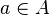# Epsilon facts for kids

Kids Encyclopedia Facts
 Quick facts for kidsGreek alphabet Other letters Αα Alpha Νν Nu Ββ Beta Ξξ Xi Γγ Gamma Οο Omicron Δδ Delta Ππ Pi Εε Epsilon Ρρ Rho Ζζ Zeta Σσς Sigma Ηη Eta Ττ Tau Θθ Theta Υυ Upsilon Ιι Iota Φφ Phi Κκ Kappa Χχ Chi Λλ Lambda Ψψ Psi Μμ Mu Ωω Omega Ϝϝ Digamma Ⱶⱶ Heta Ϻϻ San Ϙϙ Koppa Ϡϡ Sampi Ϛϛ Stigma Ϸϸ Sho

Epsilon (uppercase/lowercase Ε ε), is the letter of the Greek alphabet, used to represent the "e" sound in Ancient and Modern Greek. In the system of Greek numerals, it has a value of 5. Letters that came from it include the Roman E and Cyrillic Е. It is also used in calculus to represent a very small number (particularly in proofs involving limit). In set theory, a typographical variant of epsilon can be used to represent the statement "a is a member of set A" (symbolically$a \in A$).

(A little fact: The Ypsilon is the only letter of the alphabet that you can divide into syllables: Y-psi-lon)

## Images for kidsEpsilon Facts for Kids. Kiddle Encyclopedia.